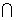Math 333/333H

Week 3

Sections 2.3-2.4

Counting Rules for Enumerating All Possible Event Outcomes
1. Product Rule: If  there are n1 possible choices for the first element of a set, n2 possible choices for the second element of a set, …., nk possible choices for the k-th element of a set, then the number of possible sets is (n1n2…nk).  (Can use tree diagram to enumerate all the possibilities)
2. Permutation Rule:  When choosing k objects from a set of n distinct objects to form an ordered sequence of size k, the number of possible sets is n(n-1)…(n-k+1)=Pk,n. Using factorial notation, we have Pk,n= n!/(n-k)!.
3. Combination Rule: When choosing k objects from a set of n distinct objects, the number of possible unordered subsets of size k is
Ck,n=  n!/[k!(n-k)!] = Pk,n/k!.
Conditional Probability

How does the  knowledge of the occurrence of one event (B) affect probability assignment to another event (A)?

Given that event B occurs, the original sample space is reduced.

Thus the conditional probability of A, given that B occurs, is defined as

P(A | B) = P( AB)/P(B)

Note: From the definition of Conditional Probability, we can get the Multiplication Rule: P(  AB) = P(A | B)P(B)